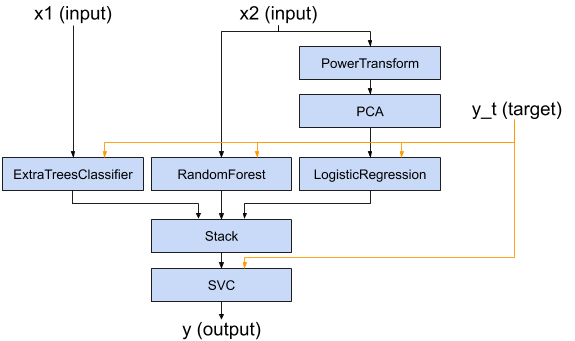A graph-based functional API for building complex scikit-learn pipelines.

## Project description

baikal is a graph-based, functional API for building complex machine learning pipelines of objects that implement the scikit-learn API. It is mostly inspired on the excellent Keras API for Deep Learning, and borrows a few concepts from the TensorFlow framework and the (perhaps lesser known) graphkit package.

baikal aims to provide an API that allows to build complex, non-linear machine learning pipelines that looks like this:with code that looks like this:

x1 = Input()
x2 = Input()
y_t = Input()

y1 = ExtraTreesClassifier()(x1, y_t)
y2 = RandomForestClassifier()(x2, y_t)
z = PowerTransformer()(x2)
z = PCA()(z)
y3 = LogisticRegression()(z, y_t)

stacked_features = Stack()([y1, y2, y3])
y = SVC()(stacked_features, y_t)

model = Model([x1, x2], y, y_t)

## Project details

This version0.4.20.4.10.4.0 yanked0.3.10.3.00.2.00.1.0

Uploaded source
Uploaded py3